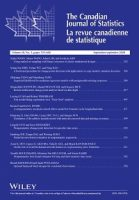# Layman’s abstract for paper on a Gaussian alternative to using improper confidence intervals

Each week, we will be publishing layman’s abstracts of new articles from our prestigious portfolio of journals in statistics. The aim is to highlight the latest research to a broader audience in an accessible format.

The article featured today is from the Canadian Journal of Statistics, with the full article now available to read in Early View here.Plante, A. (2020), A Gaussian alternative to using improper confidence intervals. Can J Statistics. doi:10.1002/cjs.11569

J. Neyman’s founding paper on confidence intervals (CIs) was published in 1937, after being refused by Egon Pearson for publication in Biometrika. It was soon criticized by A. Wald who anticipated its problematic use in many areas of estimation. A major difficulty, identified by W. G Cochran, was acknowledged by Neyman in 1948, and has been widely discussed since 1954: If a confidence belt contains unusable all inclusive CIs, the confidence coefficient is not always actually as stated, and can be significantly wrong when one uses regular CIs belonging to the same confidence belt. In fact, using uniformly most accurate or “Natural” CIs — recommended by C. Stein in 1959 as preferable to using fiducial intervals when estimating the noncentrality parameter of a noncentral chi-square distribution — provides a good example of how improper CIs arise. Stein’s CIs are even improper with probability one under certain conditions at the limit.

In this paper, both empty and all inclusive CIs are called “improper” (a term coined by H. Scheffé) since, if a one-sided CI is empty at a certain level, then the “opposite” one-sided CI is all inclusive at the complementary level. There have been many attempts to produce “improved” CIs which are consistently proper at all levels. Unfortunately, this has been unsuccessful, and proven impossible. There can be no remedy within standard CI Theory for many important interval estimation areas such as: for a ratio of means or of regression coefficients; for inverse regression and calibration problems; for finding the maximum or the minimum of a parabola; for a coefficient of variation; for the slope of a major axis of a bivariate normal distribution; for a restricted mean, and for a restricted parameter in general; for a mixture parameter; for all variance component problems; for every analysis of variance models with more than one error term; for many time series problems; for the location and concentration parameters of a distribution in circular statistics; for errors-in-variables models; etc. In 1955, R.A. Fisher suggested to J. W. Tukey that the concept of simulated observation error could be used as a means to approximately describe distributions of propagated (observation) error (DPEs). In 1957, in a paper (then classified) that few were privileged to examine, Tukey initiated a method which avoids improper CI estimation in troublesome cases. He employed quantiles of DPEs, which provide “margins of error”. This was the basis of a renewed post-Kolmogorov Gaussian Theory outlined and demonstrated in this paper.

Gauss had based his theory on a physical concept — the distribution of all the possible fortuitous errors associated with an observation. His model for a single observation can be described by the linear pivot “Observation” – “Parameter” = “Error”, where “Observation” is a number (i.e., an estimate); and, where using probability terminology, “Error” is an error variable. The associated equation “Parameter” = “Observation” – “Error” shows the “Parameter” (which can never be observed directly) as seen through an “Estimate” and an “Error”. The “Parameter” is “postfixed” by a DPE centered on this “Estimate”. When several observations are made, their associated errors are combined to give rise to a DPE on an estimate. The Gaussian error propagation formula, which provides a linear approximation to a non-linear pivot T(“Observation”;”Parameter”) = “Error” is still extensively used in the physical sciences and in engineering.

Tukey adapted Gauss’s terminology to the post-Kolmogorov era, and extended Gauss’s error propagation formula to obtain approximate DPEs. In 1961, D.A.S. Fraser’s frequency interpretation demonstrated how to observe a DPE when the pivot is linear or based on a group of transformations. This paper extends Fraser’s frequency interpretation — thus providing a method to observe DPEs in cases where the pivot is non-linear. An algorithm capable of producing a margin of error for any (computable) parameter derived from the five parameters of the bivariate normal distribution is given, as is a prototype program. Graphs demonstrate several examples, compared with CI estimates. An improved Stein’s “natural” interval estimate for a noncentrality parameter is suggested as well. It is also shown that alleged paradoxes to Fiducial Theory are invalid if attempted against a renewed Theory of Errors approach.

This renewed Theory of Errors belongs to the same extended family as do Fisher’s Fiducial Method, Fraser’s Structural Distribution Method, Dempster’s Upper and Lower Probability Method; and Hannig’s Generalized Fiducial Method. It also shares a theoretical neighborhood with Cox’s Confidence Distribution Method, and with Weerahandi’s Generalized Confidence Method.